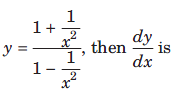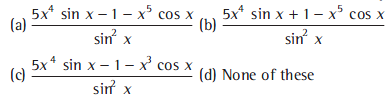# Class 11 Mathematics Limits And Derivatives MCQs

Refer to Class 11 Mathematics Limits And Derivatives MCQs provided below. CBSE Class 11 Mathematics MCQs with answers available in Pdf for free download. The MCQ Questions for Class 11 Mathematics with answers have been prepared as per the latest syllabus, CBSE books and examination pattern suggested in Class 11 by CBSE, NCERT and KVS. Multiple Choice Questions for Chapter 13 Limits and Derivatives are an important part of exams for Class 11 Mathematics and if practiced properly can help you to get higher marks. Refer to more Chapter-wise MCQs for CBSE Class 11 Mathematics and also download more latest study material for all subjects

## MCQ for Class 11 Mathematics Chapter 13 Limits and Derivatives

Class 11 Mathematics students should refer to the following multiple-choice questions with answers for Chapter 13 Limits and Derivatives in Class 11. These MCQ questions with answers for Class 11 Mathematics will come in exams and help you to score good marks

### Chapter 13 Limits and Derivatives MCQ Questions Class 11 Mathematics with Answers

Question. limx→0 sinx / √(x + 1 - √(1- x) is
(a) 2
(b) 0
(c) 1
(d) –1

Question. limx→1 (√x +1) (2x - 3) /2x3 + x -3 is
(a) 1/10
(b) 1/10
(c) 1
(d) None of these

Question. limx→4 |x-4 | /x - 4 is equal to
(a) 1
(b) –1
(c) does not exist
(d) None of these

Question. If y = √x + 1/√x , then dy/dx at x = 1 is

(a) 1
(b) 1/2
(c) 1/√2
(d) 0

Question. limx→0 |Sinx | /x  is
(a) 1
(b) –1
(c) does not exist
(d) None of these

Question. If y =(a) -4x / (x2 - 1)2

(b) -4x / (x2 - 1)
(c)  1 - x2 / 4x
(d) 4x / x2 - 1

Question. limx→0 tan2x - x / 3x - Sinx
(a) 2
(b) 1/2
(c) -1/2
(d) 1/4

Question. The derivative of (3x + 5)(1 + tan x) is
(a) 3x sec2 x 6 sec2 x 3 + 3 tan x
(b) 3x sec2 x  5 sec2 x 3 3 tan x
(c) 3x sec2 x + 5 sec2 x + 3 + 3 tan x
(d) None of the above

Question. The derivative of (ax2 + cot x)( p + q cos x) is
(a) ax2 cot (q sin ) + ( p+ q cosx )(2ax cosec2x )
(b) ax2 cot (q sin ) + ( p+ q cosx )(2ax cosec2x )
(c) ax2 cot (q sin ) + ( p q cosx )(2ax cosec2x )
(d) None of the above

Question. The derivative of (x sinx cosx) 2 + 2 is
(a) x2 cos x + 2x sin x sin 2x
(b) x2 cos x + 2x sin x sin 2x
(c) x2 cos x + x sin x sin 2x
(d) x2 cos x + 2x sin x + sin 2x

Question. The derivative of sin3x cos3x xis
(a) 3 /5 sin2 2x cos 2x
(b) 3 /4 sin2 2x cos 2x
(c) 3 /4 sin 2x cos2 2x
(d) 3 /2 sin2 2x cos 2x

Question. The derivative of x5 cos x /sinx isQuestion. Find the differentiation of x2/3 by using first principle.
(a) 2/3 x-1/3
(b) -2/3 x-1/3
(c) 2/3 x 1/3
(d) None of these

Question. Find the differentiation of cos (x2 + 1 ) by using first principle.
(a) 2x sin (x2 + 1 )
(b) 2x sin (x2 + 1 )
(c) -2x sin (x2 - 1 )
(d) None of these

Question. If  f (x )= x-4 /2√x then f '(1) is

(a) 5/4
(b) 4/5
(c) 1
(d) 0

Question. Find the differentiation of x cos x by using first principle.
(a) x sin x + cos x
(b) x sin x + cos x
(c) x cos x cos x
(d) None of these

Question. If y = Sin (x+9) / Cos x , then dy/dx at x = 0 is
(a) cos 9
(b) sin 9
(c) 0
(d) 1

 Class 11 Mathematics Set Theory MCQs
 Class 11 Mathematics Relations and Functions MCQs
 Class 11 Mathematics Trigonometric Functions MCQs
 Class 11 Mathematics Principle of Mathematical Induction Functions MCQs
 Class 11 Mathematics Complex Numbers and Quadratic Equation MCQs
 Class 11 Mathematics Linear Inequalities MCQs
 Class 11 Mathematics Permutations and Combinations MCQs
 Class 11 Mathematics Binomial Theorem MCQs
 Class 11 Mathematics Sequences and Series MCQs
 Class 11 Mathematics Straight Lines MCQs
 Class 11 Mathematics Conic Sections MCQs
 Class 11 Mathematics Introduction To Three-Dimensional Geometry MCQs
 Class 11 Mathematics Limits And Derivatives MCQs
 Class 11 Mathematics Mathematical Reasoning MCQs
 Class 11 Mathematics Statistics MCQs
 Class 11 Mathematics Probability MCQs

## More Study Material

### CBSE Class 11 Mathematics Chapter 13 Limits and Derivatives MCQs

We hope students liked the above MCQs for Chapter 13 Limits and Derivatives designed as per the latest syllabus for Class 11 Mathematics released by CBSE. Students of Class 11 should download the Multiple Choice Questions and Answers in Pdf format and practice the questions and solutions given in above Class 11 Mathematics MCQs Questions on daily basis. All latest MCQs with answers have been developed for Mathematics by referring to the most important and regularly asked topics which the students should learn and practice to get better score in school tests and examinations. Studiestoday is the best portal for Class 11 students to get all latest study material free of cost.

### MCQs for Mathematics CBSE Class 11 Chapter 13 Limits and Derivatives

Expert teachers of studiestoday have referred to NCERT book for Class 11 Mathematics to develop the Mathematics Class 11 MCQs. If you download MCQs with answers for the above chapter daily, you will get higher and better marks in Class 11 test and exams in the current year as you will be able to have stronger understanding of all concepts. Daily Multiple Choice Questions practice of Mathematics and its study material will help students to have stronger understanding of all concepts and also make them expert on all critical topics. You can easily download and save all MCQs for Class 11 Mathematics also from www.studiestoday.com without paying anything in Pdf format. After solving the questions given in the MCQs which have been developed as per latest course books also refer to the NCERT solutions for Class 11 Mathematics designed by our teachers

#### Chapter 13 Limits and Derivatives MCQs Mathematics CBSE Class 11

All MCQs given above for Class 11 Mathematics have been made as per the latest syllabus and books issued for the current academic year. The students of Class 11 can refer to the answers which have been also provided by our teachers for all MCQs of Mathematics so that you are able to solve the questions and then compare your answers with the solutions provided by us. We have also provided lot of MCQ questions for Class 11 Mathematics so that you can solve questions relating to all topics given in each chapter. All study material for Class 11 Mathematics students have been given on studiestoday.

#### Chapter 13 Limits and Derivatives CBSE Class 11 MCQs Mathematics

Regular MCQs practice helps to gain more practice in solving questions to obtain a more comprehensive understanding of Chapter 13 Limits and Derivatives concepts. MCQs play an important role in developing understanding of Chapter 13 Limits and Derivatives in CBSE Class 11. Students can download and save or print all the MCQs, printable assignments, practice sheets of the above chapter in Class 11 Mathematics in Pdf format from studiestoday. You can print or read them online on your computer or mobile or any other device. After solving these you should also refer to Class 11 Mathematics MCQ Test for the same chapter

#### CBSE MCQs Mathematics Class 11 Chapter 13 Limits and Derivatives

CBSE Class 11 Mathematics best textbooks have been used for writing the problems given in the above MCQs. If you have tests coming up then you should revise all concepts relating to Chapter 13 Limits and Derivatives and then take out print of the above MCQs and attempt all problems. We have also provided a lot of other MCQs for Class 11 Mathematics which you can use to further make yourself better in Mathematics

Where can I download latest CBSE MCQs for Class 11 Mathematics Chapter 13 Limits and Derivatives

You can download the CBSE MCQs for Class 11 Mathematics Chapter 13 Limits and Derivatives for latest session from StudiesToday.com

Can I download the MCQs of Chapter 13 Limits and Derivatives Class 11 Mathematics in Pdf

Yes, you can click on the links above and download topic wise MCQs Questions PDFs for Chapter 13 Limits and Derivatives Class 11 for Mathematics

Are the Class 11 Mathematics Chapter 13 Limits and Derivatives MCQs available for the latest session

Yes, the MCQs issued by CBSE for Class 11 Mathematics Chapter 13 Limits and Derivatives have been made available here for latest academic session

How can I download the Chapter 13 Limits and Derivatives Class 11 Mathematics MCQs

You can easily access the links above and download the Chapter 13 Limits and Derivatives Class 11 MCQs Mathematics for each topic

Is there any charge for the MCQs with answers for Class 11 Mathematics Chapter 13 Limits and Derivatives

There is no charge for the MCQs and their answers for Class 11 CBSE Mathematics Chapter 13 Limits and Derivatives you can download everything free

How can I improve my MCQs in Class 11 Mathematics Chapter 13 Limits and Derivatives

Regular revision of MCQs given on studiestoday for Class 11 subject Mathematics Chapter 13 Limits and Derivatives can help you to score better marks in exams

What are MCQs for Class 11 Mathematics Chapter 13 Limits and Derivatives

Multiple Choice Questions (MCQs) for Chapter 13 Limits and Derivatives Class 11 Mathematics are objective-based questions which provide multiple answer options, and students are required to choose the correct answer from the given choices.